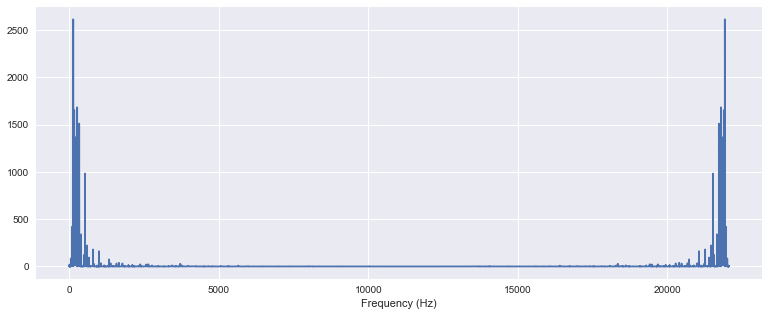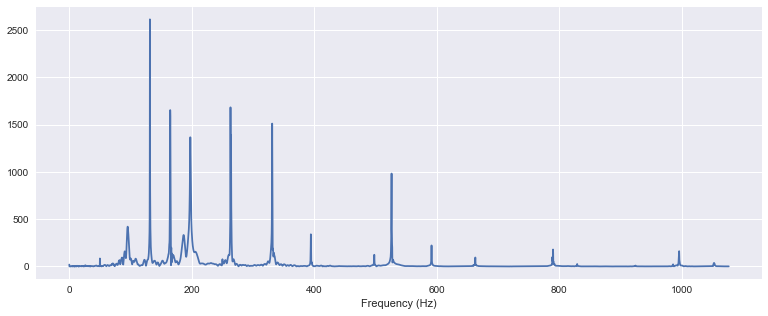In :
%matplotlib inline
import seaborn
import numpy, scipy, matplotlib.pyplot as plt, librosa, IPython.display as ipd


# Fourier TransformÂ¶

In :
import urllib
filename = 'c_strum.wav'
urllib.urlretrieve('http://audio.musicinformationretrieval.com/c_strum.wav', filename=filename)

In :
print x.shape
print sr

(102400,)
22050


Listen to the audio file:

In :
ipd.Audio(x, rate=sr)

Out:

## Fourier TransformÂ¶

The Fourier Transform (Wikipedia) is one of the most fundamental operations in applied mathematics and signal processing.

It transforms our time-domain signal into the frequency domain. Whereas the time domain expresses our signal as a sequence of samples, the frequency domain expresses our signal as a superposition of sinusoids of varying magnitudes, frequencies, and phase offsets.

To compute a Fourier transform in NumPy or SciPy, use scipy.fft:

In :
X = scipy.fft(x)
X_mag = numpy.absolute(X)
f = numpy.linspace(0, sr, len(X_mag)) # frequency variable


Plot the spectrum:

In :
plt.figure(figsize=(13, 5))
plt.plot(f, X_mag) # magnitude spectrum
plt.xlabel('Frequency (Hz)')

Out:
<matplotlib.text.Text at 0x115d84fd0>Zoom in:

In :
plt.figure(figsize=(13, 5))
plt.plot(f[:5000], X_mag[:5000])
plt.xlabel('Frequency (Hz)')

Out:
<matplotlib.text.Text at 0x11684fe10>